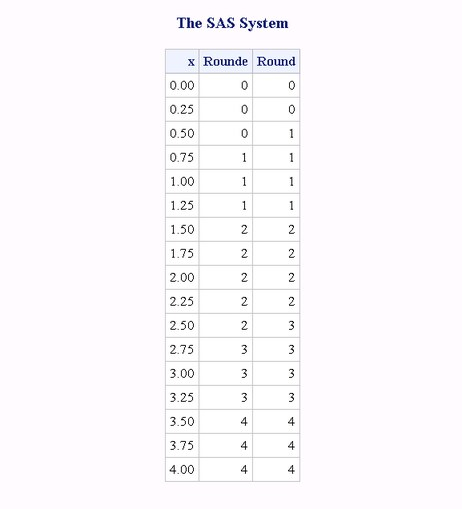# ROUNDE Function

Rounds the first argument to the nearest multiple of the second argument, and returns an even multiple when the first argument is halfway between the two nearest multiples.

 Category: Truncation

## Syntax

ROUNDE (argument )

### Required Argument

#### argument

is a numeric constant, variable, or expression to be rounded.

### Optional Argument

#### rounding-unit

is a positive, numeric constant, variable, or expression that specifies the rounding unit.

## Details

The ROUNDE function rounds the first argument to the nearest multiple of the second argument. If you omit the second argument, ROUNDE uses a default value of 1 for rounding-unit.

## Comparisons

The ROUND, ROUNDE, and ROUNDZ functions are similar with four exceptions:
• ROUND returns the multiple with the larger absolute value when the first argument is approximately halfway between the two nearest multiples of the second argument.
• ROUNDE returns an even multiple when the first argument is approximately halfway between the two nearest multiples of the second argument.
• ROUNDZ returns an even multiple when the first argument is exactly halfway between the two nearest multiples of the second argument.
• When the rounding unit is less than one and not the reciprocal of an integer, the result that is returned by ROUNDZ might not agree exactly with the result from decimal arithmetic. ROUND and ROUNDE perform extra computations, called fuzzing, to try to make the result agree with decimal arithmetic in the most common situations. ROUNDZ does not fuzz the result.

## Example

The following example compares the results that are returned by the ROUNDE function with the results that are returned by the ROUND function.
```data results;
do x=0 to 4 by .25;
Rounde=rounde(x);
Round=round(x);
output;
end;
run;
proc print data=results noobs;
run;```
Results That are Returned by the ROUNDE and ROUND Functions